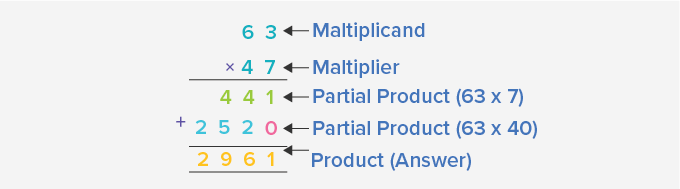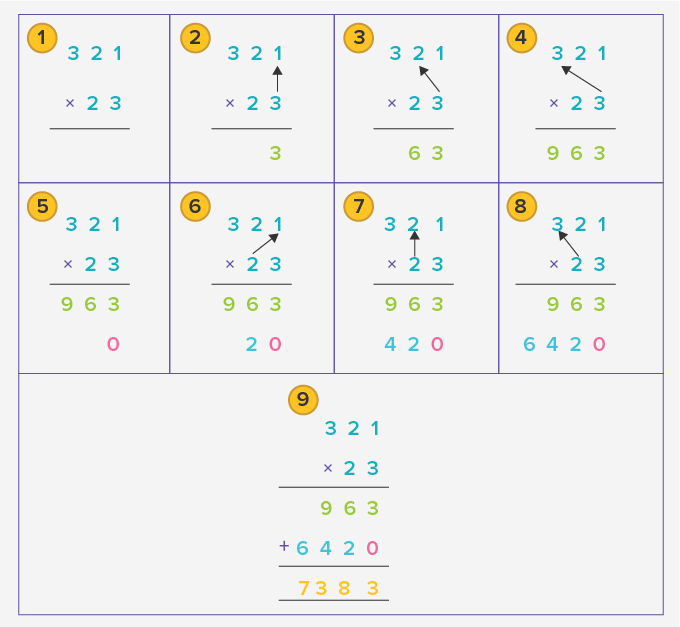Long Multiplication - Definition with Examples

The Complete K-5 Math Learning Program Built for Your Child

• 30 Million Kids

Loved by kids and parent worldwide

• 50,000 Schools

Trusted by teachers across schools

• Comprehensive Curriculum

Aligned to Common Core

Long Multiplication

Long multiplication is a method of multiplying two numbers which are difficult to multiply easily.

For example, we can easily find the product of 55 × 20 by multiplying 55 by 2 and then adding a 0 at the rightmost place of the answer.

55 × 2 = 110 and 55 × 20 = 1100.

But, many a time finding the product is not this easy. At such times we use the long method of multiplication.

Steps to multiply using Long Multiplication

Multiplying 2-Digit by 2-Digit Numbers

Let us multiply 47 by 63 using the long multiplication method.

1. Write the two numbers one below the other as per the places of their digits. Write the bigger number on top and a multiplication sign on the left. Draw a line below the numbers.2. Multiply ones digit of the top number by the ones digit of the bottom number.

Write the product as shown.3. Multiply the tens digit of the top number by the ones digit of the bottom number.This is our first partial product which we got on multiplying the top number by the ones digit of the bottom number.

4. Write a 0 below the ones digit as shown. This is because we will now be multiplying the digits of the top number by the tens digit of the bottom number. Hence, we write a 0 in the ones place.5. Multiply the ones digit of the top number by the tens digit of the bottom number.6. Multiply the tens digit of the top number by the tens digit of the bottom number.This is the second partial product obtained on multiplying the top number by the tens digit of the bottom number.

7. Add the two partial products.In long multiplication method, the number on the top is called the multiplicand. The number by which it is multiplied, that is, the bottom number is called the multiplier.

So, a long division problem will have:We follow the same method for multiplying numbers greater than 2-Digits.

The figure below shows the long division method to multiply 357 by 23Fun Fact: Long multiplication is also known as column method of multiplication.

Won Numerous Awards & Honors